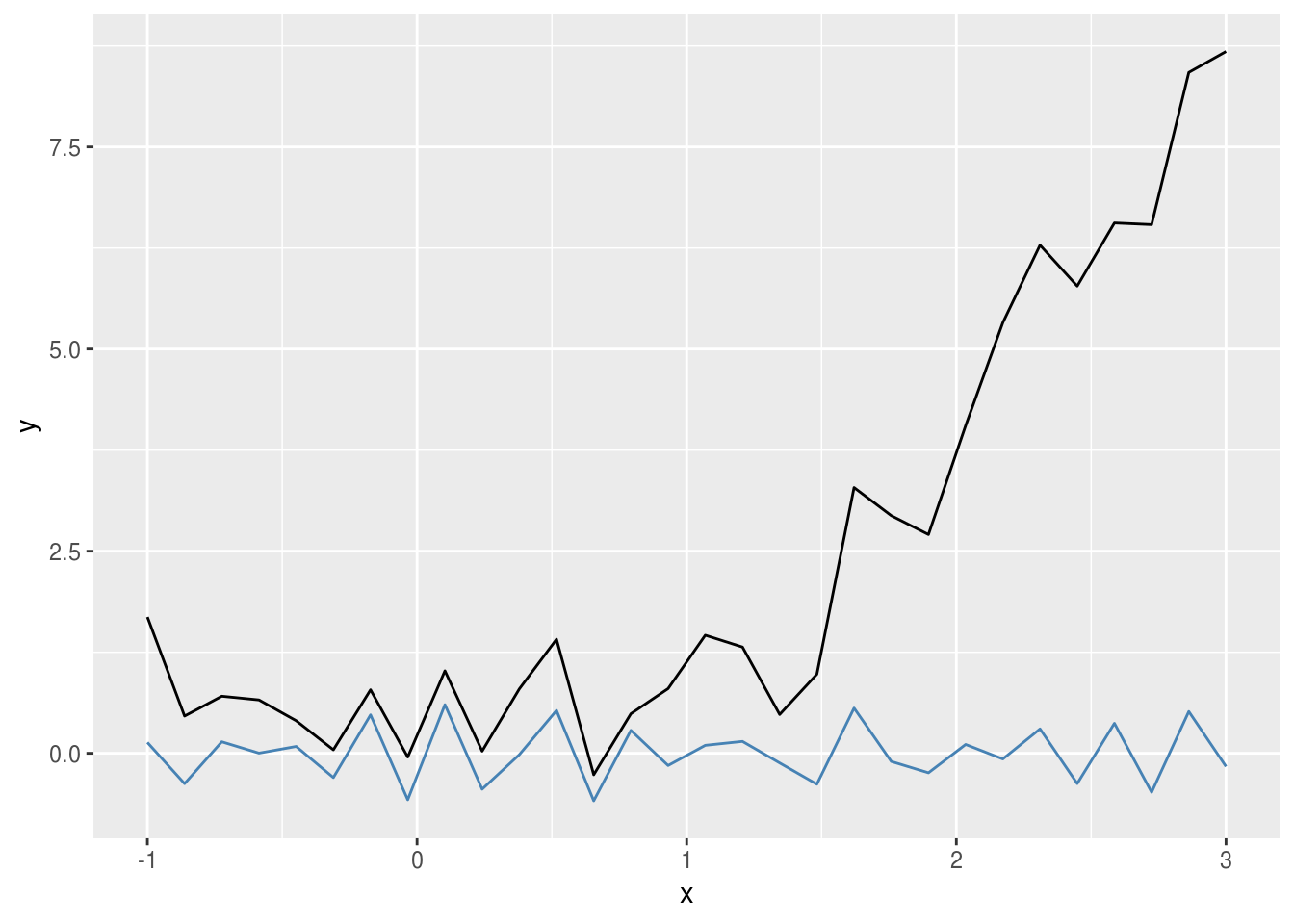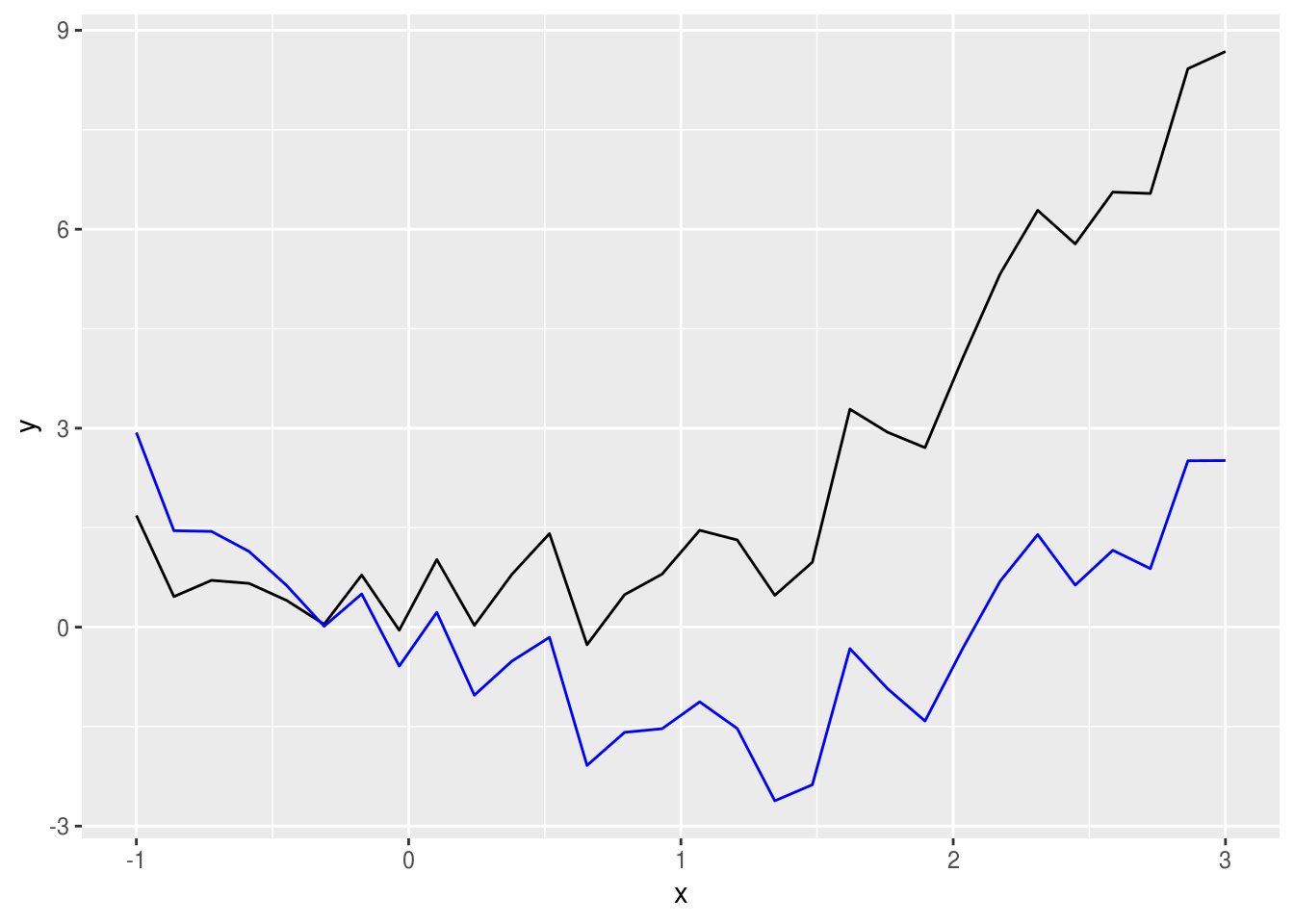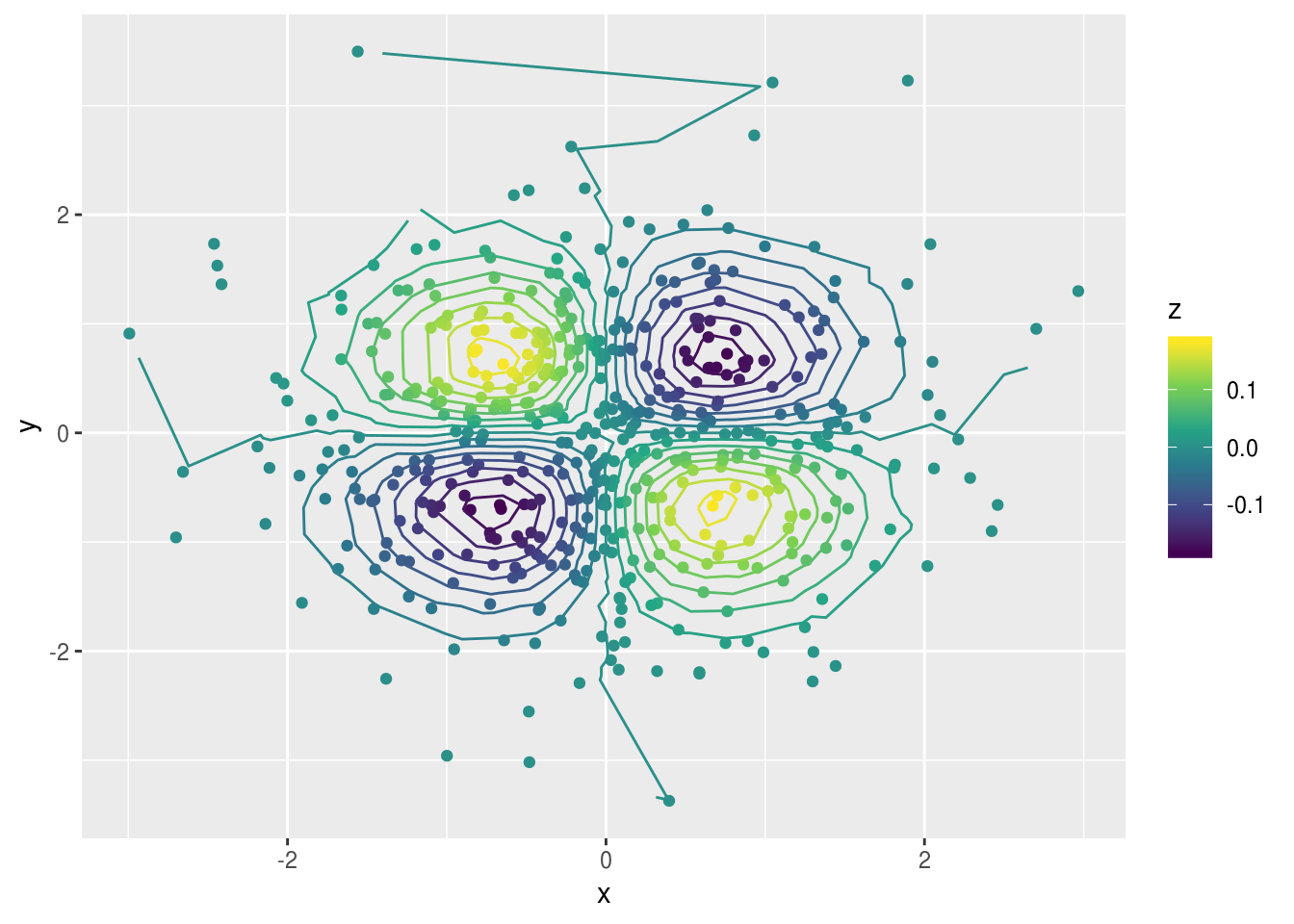For a while now I’ve been thinking that, yes, ggplot2 is awesome and offers a lot of geoms and stats, but it would be great if it could be extended with new user-generated geoms and stats. Then I learnt that ggplot2 actually has a pretty great extension system so I could create my own geoms I needed for my work or just for fun. But still, creating a geom from scratch is an involved process that doesn’t lend itself to simple transformations.

Finally, I thought of a possible solution: create a generic stat –a tabula rasa, if you will– that can work on the data with any function. Natively ggplot2 offers stat_summary(), but it’s only meant to be used with, well, summary statistics. What I wanted was something completely generic and this is my first try.

Below is the code for stat_rasa() (better name pending). It works just like any other stat except that it works with any function that takes a data.frame and returns a transformed data.frame that can be interpreted by the chosen geom.

# ggproto object
StatRasa <- ggplot2::ggproto("StatRasa", ggplot2::Stat,
compute_group = function(data, scales, fun, fun.args) {
# Change default arguments of the function to the
# values in fun.args
args <- formals(fun)
for (i in seq_along(fun.args)) {
if (names(fun.args[i]) %in% names(fun.args)) {
args[[names(fun.args[i])]] <- fun.args[[i]]
}
}
formals(fun) <- args

# Apply function to data
fun(data)
})

# stat function used in ggplot
stat_rasa <- function(mapping = NULL, data = NULL,
geom = "point",
position = "identity",
fun = NULL,
...,
show.legend = NA,
inherit.aes = TRUE) {
# Check arguments
if (!is.function(fun)) stop("fun must be a function")

# Pass dotted arguments to a list
fun.args <- match.call(expand.dots = FALSE)$... ggplot2::layer( data = data, mapping = mapping, stat = StatRasa, geom = geom, position = position, show.legend = show.legend, inherit.aes = inherit.aes, check.aes = FALSE, check.param = FALSE, params = list( fun = fun, fun.args = fun.args, na.rm = FALSE, ... ) ) }  For example, let’s say we want to quickly glance at detrended data. We then create a very simple function Detrend <- function(data, method = "lm", span = 0.2) { if (method == "lm") { data$y <- resid(lm(y ~ x, data = data))
} else {
data$y <- resid(loess(y ~ x, span = span, data = data)) } as.data.frame(data) }  and pass it to stat_rasa() library(ggplot2) set.seed(42) x <- seq(-1, 3, length.out = 30) y <- x^2 + rnorm(30)*0.5 df <- data.frame(x = x, y = y) ggplot(df, aes(x, y)) + geom_line() + stat_rasa(geom = "line", fun = Detrend, method = "smooth", color = "steelblue")We can get better legibility and less typing by creating a wrapper function with a more descriptive name. stat_detrend <- function(...) { stat_rasa(fun = Detrend, ...) } ggplot(df, aes(x, y)) + geom_line() + stat_detrend(method = "lm", color = "blue", geom = "line")Another case could be calculating contours from an irregular grid. Since ggplot2::stat_contour() uses grDevices::contourLines(), it needs values defined in a regular grid, but there’s a package called contoureR that can compute contours from irregularly spaced observations. With stat_rasa() we can integrate it with ggplot2 effortlessly by creating a small function and using geom = "path". IrregularContour <- function(data, breaks = scales::fullseq, binwidth = NULL, bins = 10) { if (is.function(breaks)) { # If no parameters set, use pretty bins to calculate binwidth if (is.null(binwidth)) { binwidth <- diff(range(data$z)) / bins
}

breaks <- breaks(range(data$z), binwidth) } cl <- contoureR::getContourLines(x = data$x, y = data$y, z = data$z,
levels = breaks)

if (length(cl) == 0) {
warning("Not possible to generate contour data", call. = FALSE)
return(data.frame())
}
cl <- cl[, 3:7]
colnames(cl) <- c("piece", "group", "x", "y", "level")
return(cl)
}

stat_contour_irregular <- function(...) {
stat_rasa(fun = IrregularContour, geom = "path", ...)
}

set.seed(42)
df <- data.frame(x = rnorm(500),
y = rnorm(500))
df\$z <- with(df, -x*y*exp(-x^2 - y^2))

ggplot(df, aes(x, y)) +
geom_point(aes(color = z)) +
stat_contour_irregular(aes(z = z, color = ..level..), bins = 15) +
scale_color_viridis_c()And voilà.

There’s always things to improve. For example, the possibility of using a custom function to compute parameters that depend on the data, but I believe that as it stands covers 80% of simple applications. I should also use a better name, but naming things is hard work.